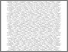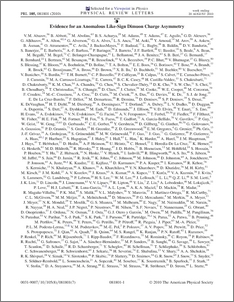# Evidence for an anomalous like-sign dimuon charge asymmetry

Collaboration, D0 and Bertram, Iain and Borissov, Guennadi and Fox, Harald and Williams, Mark and Ratoff, Peter and Love, Peter (2010) Evidence for an anomalous like-sign dimuon charge asymmetry. Physical review letters, 105 (8). ISSN 1079-7114Preview
PDF
PhysRevLett.105.081801.pdf - Published Version

We measure the charge asymmetry $A\equiv(N^{++} - N^{--})/(N^{++} + N^{--})$ of like-sign dimuon events in 6.1~fb$^{-1}$ of $p\bar{p}$ collisions recorded with the D0 detector at a center-of-mass energy $\sqrt{s}=1.96$~TeV at the Fermilab Tevatron collider. From $A$ we extract the like-sign dimuon charge asymmetry in semileptonic $b$-hadron decays: $\aslb=-0.00957\pm0.00251\thinspace({\rm stat})\pm0.00146\thinspace({\rm sys})$. It differs by 3.2~standard deviations from the standard model prediction $\aslb({\rm SM})=(-2.3^{+0.5}_{-0.6})\times10^{-4}$, and provides first evidence of anomalous $CP$ violation in the mixing of neutral $B$ mesons.View Item